# Basic Multiplication (0-10)

Times up!
Times up! You may finish answering the current question and then proceed to your score!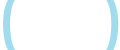Sorry, you have used all of your available hints for this lesson.
Practice Limit Reached

You've reached your daily practice limit of 12 questions.

Award InformationAwarded toQuestion Hint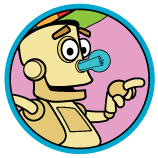Choose Family Member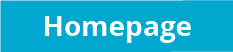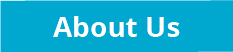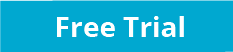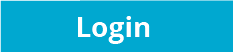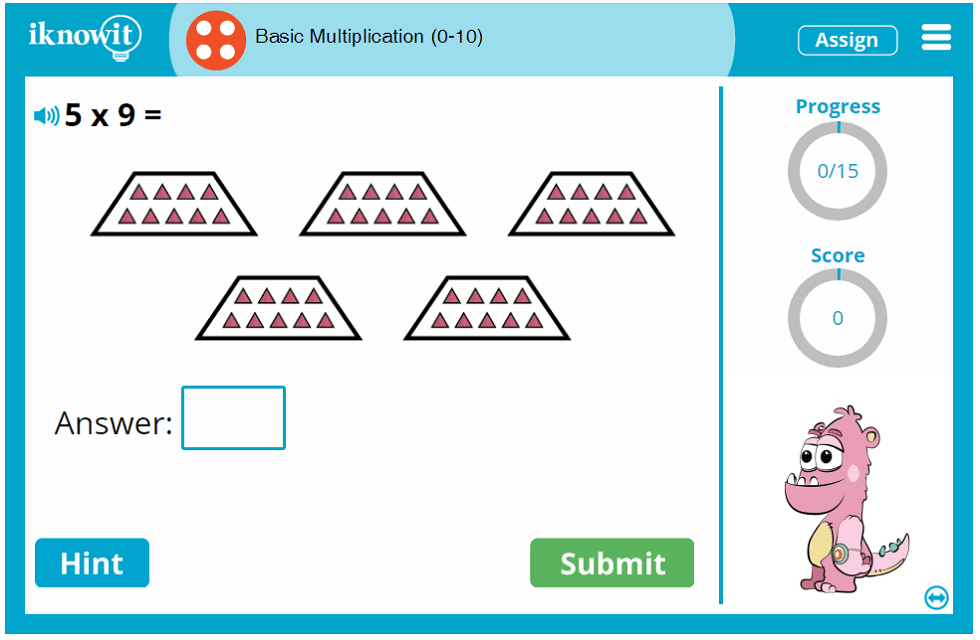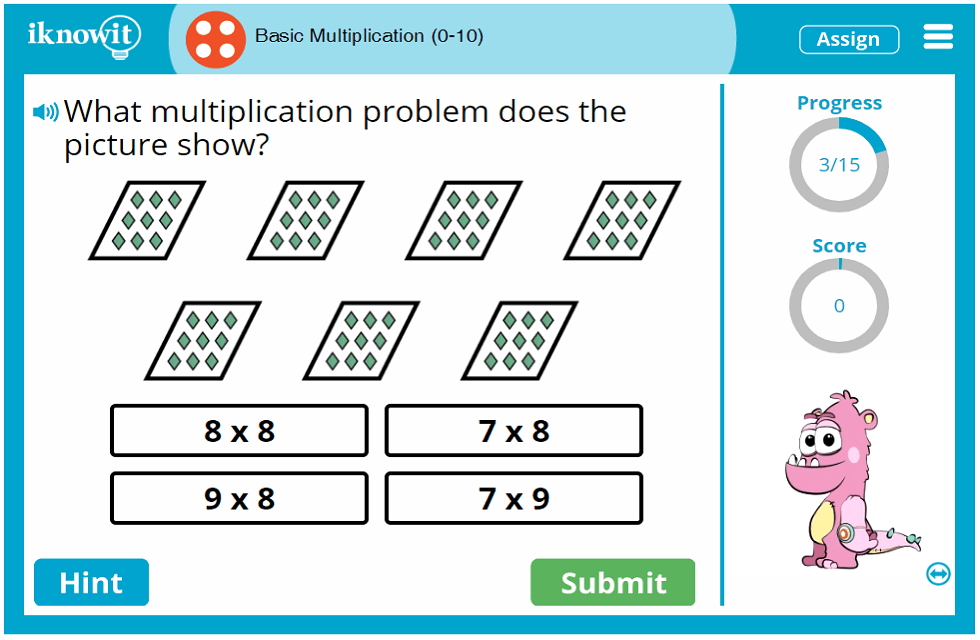## Interactive Math Lesson - Basic Multiplication from 0 to 10

If you're looking for an opportunity to practice basic multiplication skills with your fourth grade students, then try out this multiplication lesson! Your students will practice multiplication with factors from zero to ten. Multiplication problems are presented in a variety of formats: vertical multiplication problems, horizontal multiplication problems, and word problems. When students finish this interactive math game, they will be more confident and proficient in their basic multiplication skills.

Many of the math problems in this interactive multiplication lesson include visual aids, like multiplication arrays. When students need a little extra help solving the math problem, a hint button is available for them to click. For example, if students are given a word problem: "Lorenzo is filling candy bags for his 3 friends. If he puts 4 lollipops in each bag, how many lollipops will he have in all?" students can click the hint button, which will show them "3 x 4 = ?" If students answer the math problem incorrectly, a detailed explanation will appear to show them how the multiplication problem should be solved. When they move through the lesson, they will learn from past mistakes.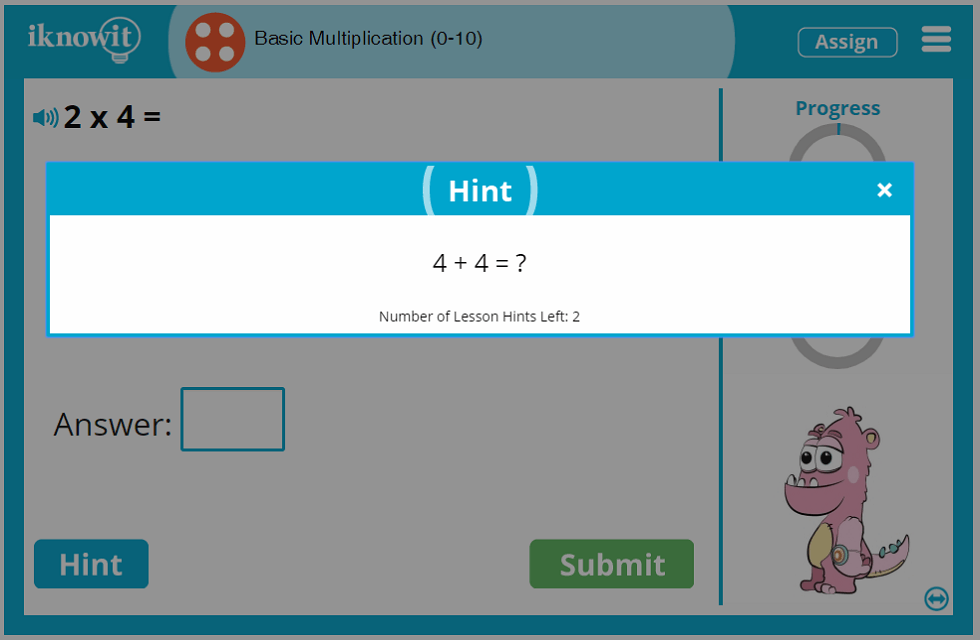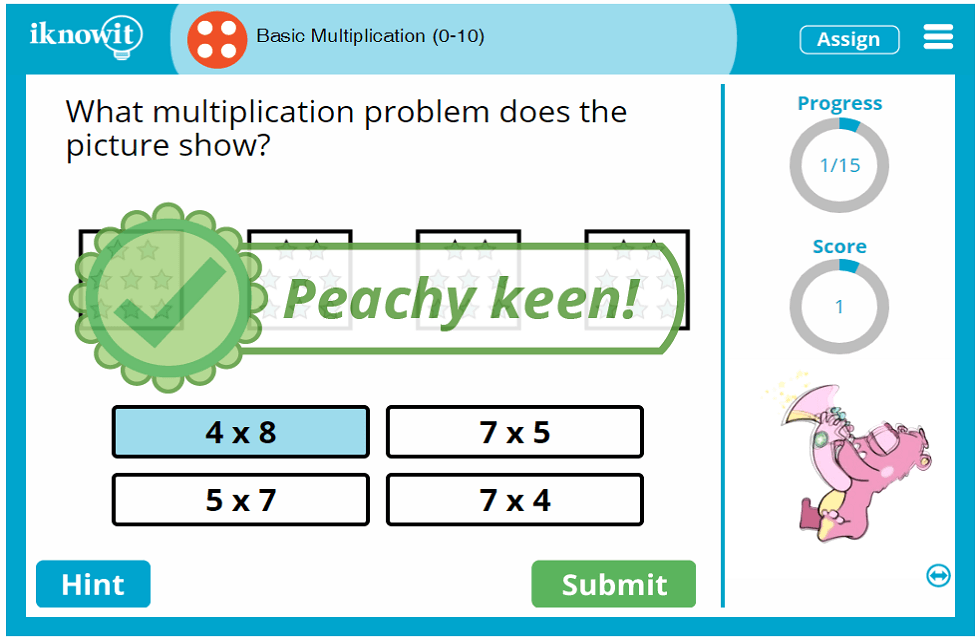## Helpful Features in I Know It Math Lessons

In addition to the availability of hints and detailed explanations, this interactive multiplication game offers several other features to help students make the most out of their math practice session. For example, a progress-tracker on the right-hand side of the practice screen lets students know how many questions are left to answer in the math lesson. A score-tracker beneath that tells students how many questions they've answered correctly so far in the lesson. A speaker icon in the upper-left corner of the screen provides a read-aloud option for ESL/ELL students, and for students who prefer auditory learning.

We hope that when you try this basic multiplication lesson from 0 to 10, you will enjoy the kid-friendly lesson format, bright and colorful icons, and whimsical animated characters. In fact, we encourage you to explore the hundreds of >other math topics available on 'I Know It' today!

Sign up for a free sixty-day trial of iKnowIt.com and try out this basic multiplication lesson today at no cost! Your class may practice for free, but please know they will be limited to a total of twenty-five math problems across all math lessons on 'I Know It' per day. For unlimited access to 'I Know It' and all its features, you will need to become a paying member of the website.

When you subscribe to 'I Know It,' your membership will unlock the website's administrative features, allowing you to create a class roster and add your students to it, assign specific lessons to individual students, track student progress and lesson scores, print, send, and download student progress reports, and change lesson settings (including turning off the animations and limiting lesson hints).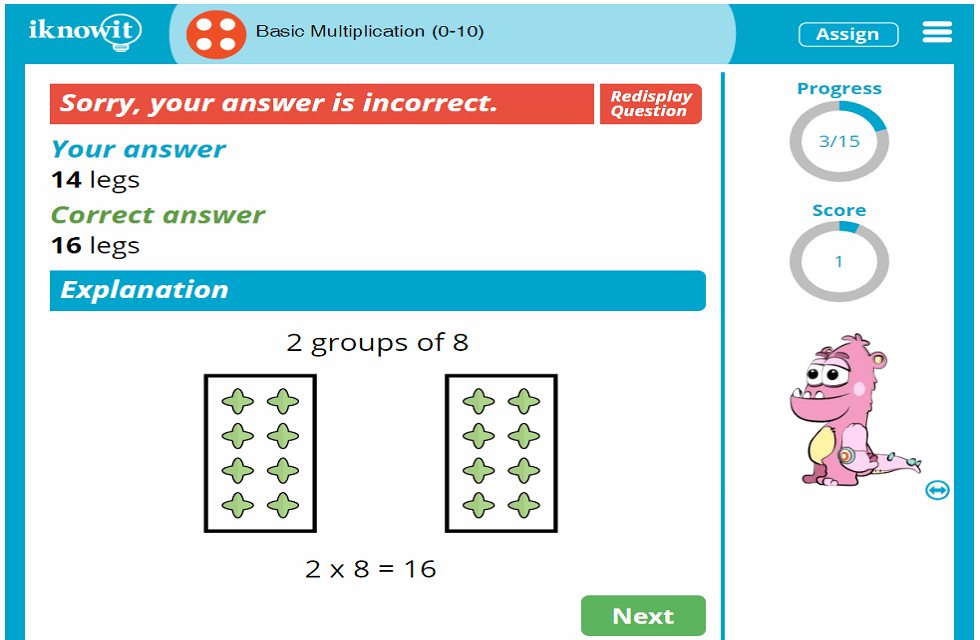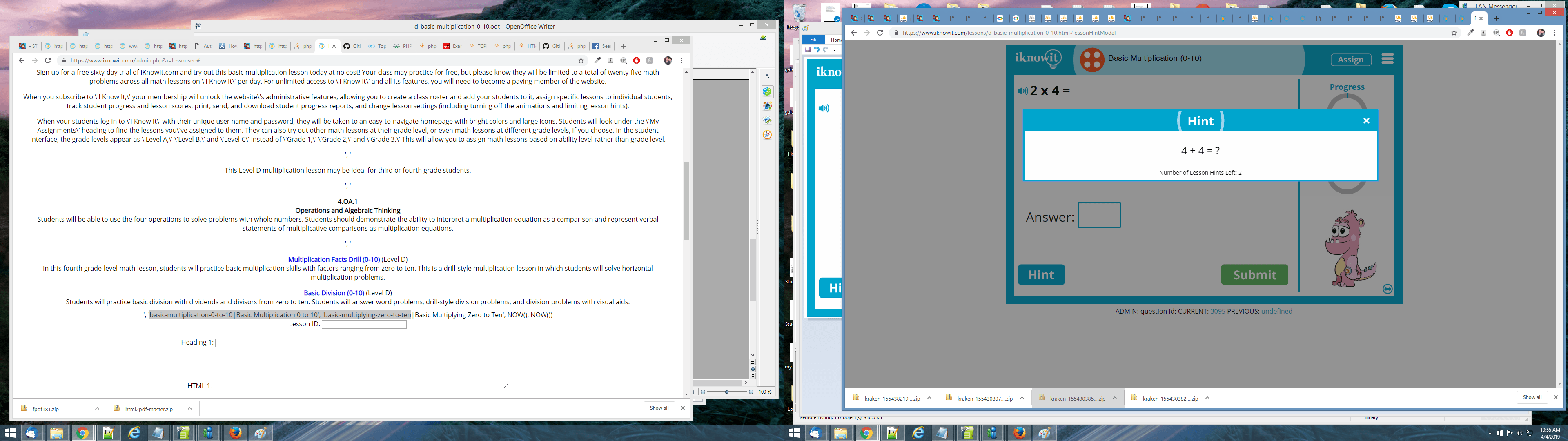## Level

This Level D multiplication lesson may be ideal for third or fourth grade students.

## Common Core Standard

4.OA.1
Operations and Algebraic Thinking

Students will be able to use the four operations to solve problems with whole numbers. Students should demonstrate the ability to interpret a multiplication equation as a comparison and represent verbal statements of multiplicative comparisons as multiplication equations.

## You might also be interested in...

Multiplication Facts Drill (0-10) (Level D)
In this fourth grade-level math lesson, students will practice basic multiplication skills with factors ranging from zero to ten. This is a drill-style multiplication lesson in which students will solve horizontal multiplication problems.

Basic Division (0-10) (Level D)
Students will practice basic division with dividends and divisors from zero to ten. Students will answer word problems, drill-style division problems, and division problems with visual aids.